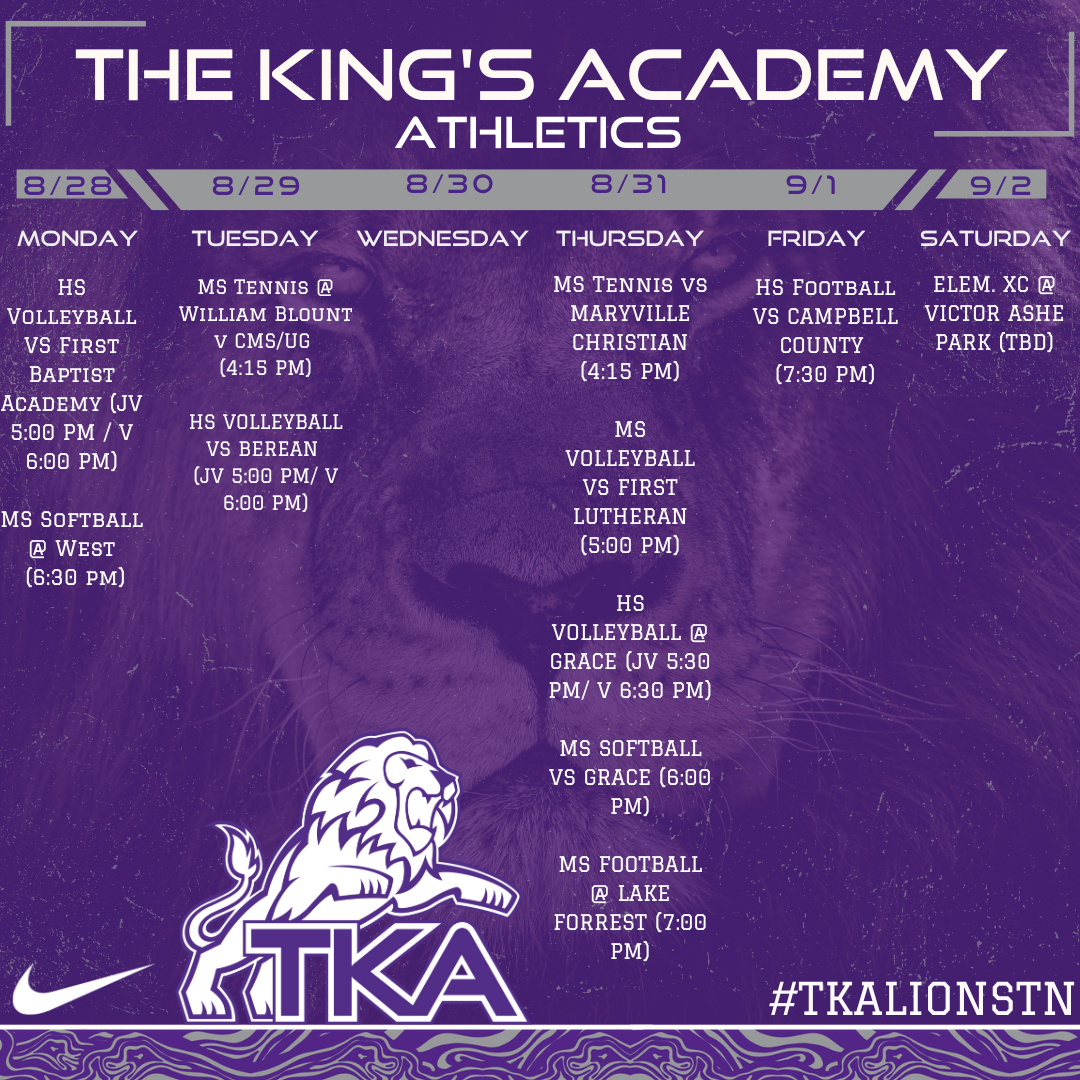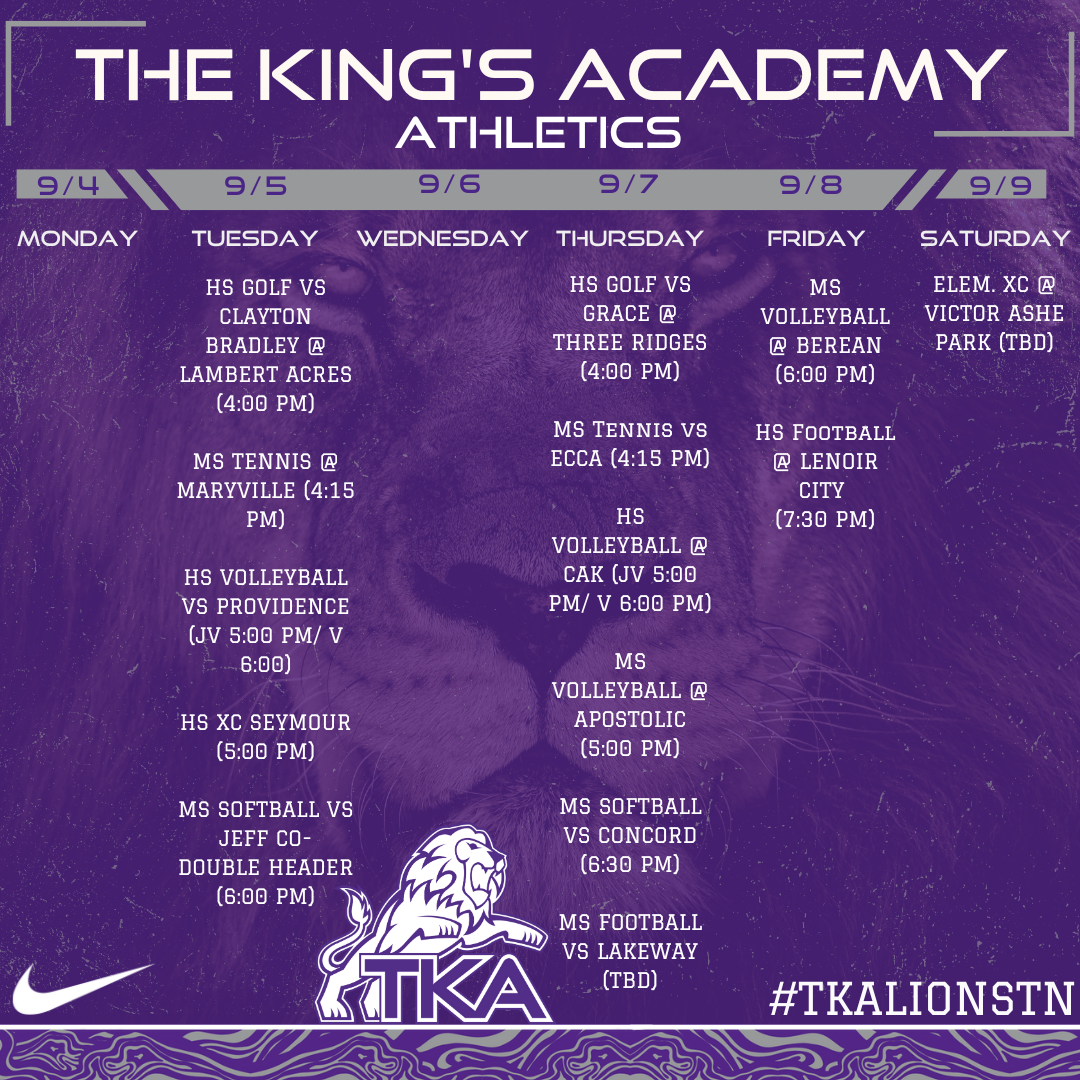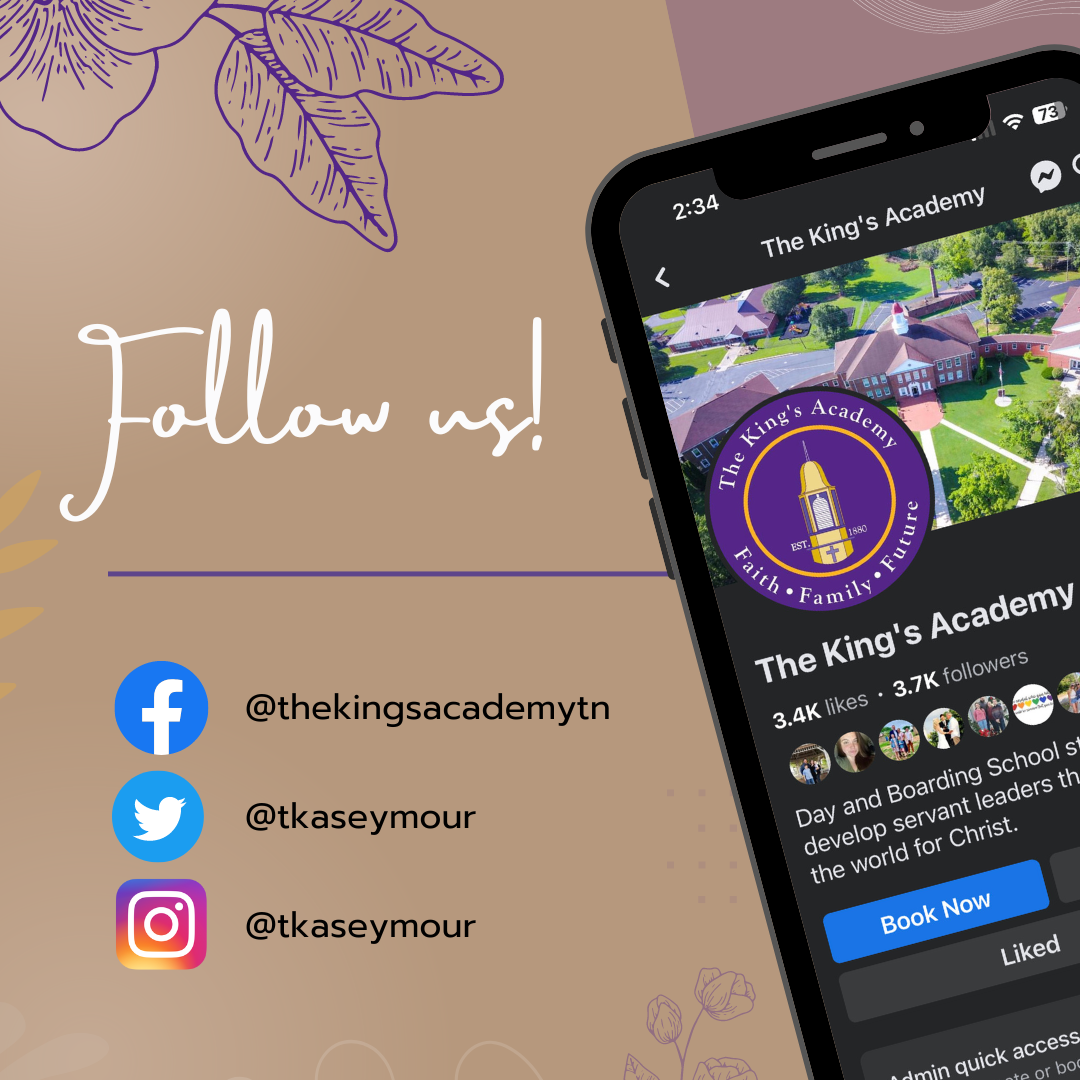## Lions Weekly: August 30, 2023# A Note From Mr. Sandefur

Lion Family,

Greetings as we embark on another week of exploration and growth at The King's Academy. I trust that each day has been a stepping stone towards progress and discovery for our students. As we navigate the journey of education together, let us turn our attention to the profound significance of being "Effective Communicators."

In Psalms 19:14, we find the prayer, "Let the words of my mouth and the meditation of my heart be acceptable in your sight, O Lord, my rock and my redeemer." This verse serves as a reminder that our words hold great power and influence, not only in our personal lives but also within our wider community. At The King's Academy, we nurture the development of effective communicators who understand the weight of their words and the impact they can have on those around them.

To be an effective communicator means more than just speaking eloquently; it requires the ability to convey thoughts, ideas, and emotions with empathy and clarity. As we instill this quality in our students, we're nurturing their capacity to create connections, foster understanding, and promote unity. Let's encourage them to embrace the power of thoughtful communication, both in their academic pursuits and in their relationships, as they navigate their journey as servant leaders of Christ.

May our students be guided by the spirit of Psalms 19:14, making their words and thoughts a reflection of the grace and wisdom that come from above. Let us all join in this endeavor to nurture effective communicators who will make a lasting impact on the world for Christ.

In Christ,

Jeremy Sandefur

 table div table+table+table+table div table{width:100%;padding:0}table div table+table+table+table div table img{width:96.23%;padding:0;float:none}table div table+table+table+table div table td{width:100%;padding:0 1.88% 18px}/* styles */table div table+table+table+table+table+table div table{width:100%;padding:0}table div table+table+table+table+table+table div table img{width:96.23%;padding:0;float:none}table div table+table+table+table+table+table div table td{width:100%;padding:0 1.88% 18px}/* styles */# Grandparents Day

Mark your calendars! Grandparents Day is just around the corner on September 8th. It's a wonderful opportunity to celebrate and cherish the bond between generations.

Please RSVP on the link below. The registration form will close at noon on Friday, September 1st. This link will also be used for parent permission for student release. The student release will allow a student’s grandparent to check them out of school following the program. On the day of the event, grandparents will sign out your child in the classroom with your child’s teacher, not the elementary office.

 table div table+table+table+table+table+table+table+table+table div table{width:100%;padding:0}table div table+table+table+table+table+table+table+table+table div table img{width:96.23%;padding:0;float:none}table div table+table+table+table+table+table+table+table+table div table td{width:100%;padding:0 1.88% 18px}/* styles */# TKA Junior League!

Is your 2nd - 5th grader interested in playing basketball for TKA's Jr. League teams this year? Please complete this brief 'TKA Jr. League Basketball Pre-Registration Form' that will assist us in determining interest and developing preliminary rosters. Once completed, our Junior League leadership team will evaluate the responses and reach out to you with additional information.

Jr. League is a competitive elementary basketball league which typically consists of 1-2 practices per week beginning in September. Games / tournaments begin in early November in gyms throughout Sevier County (TKA included) and last through early-February.

We look forward to hearing from you!

tkahoops@gmail.com

 table div table+table+table+table+table+table+table+table+table+table+table+table div table{width:100%;padding:0}table div table+table+table+table+table+table+table+table+table+table+table+table div table img{width:96.23%;padding:0;float:none}table div table+table+table+table+table+table+table+table+table+table+table+table div table td{width:100%;padding:0 1.88% 18px}/* styles */# Home Athletic Ticket Purchases

Get ready for an exciting season of TKA athletics this fall! We're thrilled to offer a convenient way to purchase your tickets for all our home events. The GoFan link below provides options for season tickets covering all our fall sports, as well as individual tickets for specific games. As part of our efforts to provide a safe and streamlined experience, we're aiming for cashless transactions at the gates. Please share these links with your families and friends to help ensure a smooth entry to our games. Remember, all TKA students get in for free. If you have any questions, don't hesitate to reach out.
-Coach Jones

 table div table+table+table+table+table+table+table+table+table+table+table+table+table+table+table div table{width:100%;padding:0}table div table+table+table+table+table+table+table+table+table+table+table+table+table+table+table div table img{width:96.23%;padding:0;float:none}table div table+table+table+table+table+table+table+table+table+table+table+table+table+table+table div table td{width:100%;padding:0 1.88% 18px}/* styles */# Legacy Matters: Conversations on Faith, Family, and Future

Exciting news! This week we launched our new podcast, "Legacy Matters: Conversations on Faith, Family, and Future." Tune in to hear engaging discussions with our school leaders, guests, and students as we explore the values that define us – faith, family, and the journey towards a purposeful future. Join us on platforms such as Spotify, Apple Podcasts, and more. Don't miss out on insightful conversations that echo the heart of The King's Academy. Follow the links below to tune in on all major podcast platforms to be part of these enriching discussions that shape our path towards developing servant leaders that will change the world for Christ. Your support and engagement means the world to us!

## Listen at the links below!

Apple Podcasts

Spotify

More

 table div table+table+table+table+table+table+table+table+table+table+table+table+table+table+table+table+table div table{width:100%;padding:0}table div table+table+table+table+table+table+table+table+table+table+table+table+table+table+table+table+table div table img{width:96.23%;padding:0;float:none}table div table+table+table+table+table+table+table+table+table+table+table+table+table+table+table+table+table div table td{width:100%;padding:0 1.88% 18px}/* styles */# "Rope in a Win!"

Friday is Spirit Day! Put on your best Western gear and "rope in a win" with us. Let's wrangle up some school spirit and have a rootin' tootin' good time! Remember, all attire must adhere to dress code!

 table div table+table+table+table+table+table+table+table+table+table+table+table+table+table+table+table+table+table+table div table{width:100%;padding:0}table div table+table+table+table+table+table+table+table+table+table+table+table+table+table+table+table+table+table+table div table img{width:96.23%;padding:0;float:none}table div table+table+table+table+table+table+table+table+table+table+table+table+table+table+table+table+table+table+table div table td{width:100%;padding:0 1.88% 18px}/* styles */# Book Fair Volunteers Needed!

The TKA Book Fair is just around the corner. Mrs. Durham is in need of volunteers to help set up, to help Little Lions shop, to help with the cash register, and to help take down. If you would be willing to help, please sign up using the link below. Volunteers are needed Thursday, September 7 - Friday, September 15.

The book fair will begin on Grandparents Day (September 8) and run through the following week (September 15). Look for more information about the fair in next week's email.

 table div table+table+table+table+table+table+table+table+table+table+table+table+table+table+table+table+table+table+table+table+table+table div table{width:100%;padding:0}table div table+table+table+table+table+table+table+table+table+table+table+table+table+table+table+table+table+table+table+table+table+table div table img{width:96.23%;padding:0;float:none}table div table+table+table+table+table+table+table+table+table+table+table+table+table+table+table+table+table+table+table+table+table+table div table td{width:100%;padding:0 1.88% 18px}/* styles */Calling all compassionate hearts! If you're interested in making a meaningful difference in the lives of our Residence Life students, we invite you to join our Residence Life Facebook Page. Whether you're a parent, staff member, or community member, your support matters. Stay updated on opportunities to mentor, provide encouragement, or contribute to their well-being. Together, we can create a warm and nurturing environment that helps our students thrive. Join us on this journey of impact and transformation!

 table div table+table+table+table+table+table+table+table+table+table+table+table+table+table+table+table+table+table+table+table+table+table+table+table+table div table{width:100%;padding:0}table div table+table+table+table+table+table+table+table+table+table+table+table+table+table+table+table+table+table+table+table+table+table+table+table+table div table img{width:96.23%;padding:0;float:none}table div table+table+table+table+table+table+table+table+table+table+table+table+table+table+table+table+table+table+table+table+table+table+table+table+table div table td{width:100%;padding:0 1.88% 18px}/* styles */## Homecoming Information!# TURN UP THE SPIRIT!

Get ready to ignite the school spirit as we announce this year's thrilling Homecoming theme: "Turn Up the Spirit!" Prepare for an unforgettable week of festivities, camaraderie, and celebration of our Lion spirit our Lion pride like never before.

 table div table+table+table+table+table+table+table+table+table+table+table+table+table+table+table+table+table+table+table+table+table+table+table+table+table+table+table+table+table div table{width:100%;padding:0}table div table+table+table+table+table+table+table+table+table+table+table+table+table+table+table+table+table+table+table+table+table+table+table+table+table+table+table+table+table div table img{width:96.23%;padding:0;float:none}table div table+table+table+table+table+table+table+table+table+table+table+table+table+table+table+table+table+table+table+table+table+table+table+table+table+table+table+table+table div table td{width:100%;padding:0 1.88% 18px}/* styles */## Aug. 28-Sept. 9 Sports Scheduletable div table+table+table+table+table+table+table+table+table+table+table+table+table+table+table+table+table+table+table+table+table+table+table+table+table+table+table+table+table+table+table+table+table div table{width:100%;padding:0}table div table+table+table+table+table+table+table+table+table+table+table+table+table+table+table+table+table+table+table+table+table+table+table+table+table+table+table+table+table+table+table+table+table div table img{width:96.23%;padding:0;float:none}table div table+table+table+table+table+table+table+table+table+table+table+table+table+table+table+table+table+table+table+table+table+table+table+table+table+table+table+table+table+table+table+table+table div table td{width:100%;padding:0 1.88% 18px}/* styles */table div table+table+table+table+table+table+table+table+table+table+table+table+table+table+table+table+table+table+table+table+table+table+table+table+table+table+table+table+table+table+table+table+table+table+table+table+table div table{width:100%;padding:0}table div table+table+table+table+table+table+table+table+table+table+table+table+table+table+table+table+table+table+table+table+table+table+table+table+table+table+table+table+table+table+table+table+table+table+table+table+table div table img{width:96.23%;padding:0;float:none}table div table+table+table+table+table+table+table+table+table+table+table+table+table+table+table+table+table+table+table+table+table+table+table+table+table+table+table+table+table+table+table+table+table+table+table+table+table div table td{width:100%;padding:0 1.88% 18px}/* styles */# Instructional Calendar

 table div table+table+table+table+table+table+table+table+table+table+table+table+table+table+table+table+table+table+table+table+table+table+table+table+table+table+table+table+table+table+table+table+table+table+table+table+table+table+table+table div table td,table.module-39{width:100%;padding:0}table div table+table+table+table+table+table+table+table+table+table+table+table+table+table+table+table+table+table+table+table+table+table+table+table+table+table+table+table+table+table+table+table+table+table+table+table+table+table+table+table div table{width:100%;float:none;margin-left:auto;margin-right:auto;padding:0}table div table+table+table+table+table+table+table+table+table+table+table+table+table+table+table+table+table+table+table+table+table+table+table+table+table+table+table+table+table+table+table+table+table+table+table+table+table+table+table+table div table a{border:0 none;text-decoration:none}table div table+table+table+table+table+table+table+table+table+table+table+table+table+table+table+table+table+table+table+table+table+table+table+table+table+table+table+table+table+table+table+table+table+table+table+table+table+table+table+table div table img{width:100%!important;border:0 none;text-decoration:none}/* styles */

# Dress Code

 table div table+table+table+table+table+table+table+table+table+table+table+table+table+table+table+table+table+table+table+table+table+table+table+table+table+table+table+table+table+table+table+table+table+table+table+table+table+table+table+table+table+table div table td,table.module-41{width:100%;padding:0}table div table+table+table+table+table+table+table+table+table+table+table+table+table+table+table+table+table+table+table+table+table+table+table+table+table+table+table+table+table+table+table+table+table+table+table+table+table+table+table+table+table+table div table{width:100%;float:none;margin-left:auto;margin-right:auto;padding:0}table div table+table+table+table+table+table+table+table+table+table+table+table+table+table+table+table+table+table+table+table+table+table+table+table+table+table+table+table+table+table+table+table+table+table+table+table+table+table+table+table+table+table div table a{border:0 none;text-decoration:none}table div table+table+table+table+table+table+table+table+table+table+table+table+table+table+table+table+table+table+table+table+table+table+table+table+table+table+table+table+table+table+table+table+table+table+table+table+table+table+table+table+table+table div table img{width:100%!important;border:0 none;text-decoration:none}/* styles */

## Dress Code Resources

 table div table+table+table+table+table+table+table+table+table+table+table+table+table+table+table+table+table+table+table+table+table+table+table+table+table+table+table+table+table+table+table+table+table+table+table+table+table+table+table+table+table+table+table+table+table div table{width:100%;padding:0}table div table+table+table+table+table+table+table+table+table+table+table+table+table+table+table+table+table+table+table+table+table+table+table+table+table+table+table+table+table+table+table+table+table+table+table+table+table+table+table+table+table+table+table+table+table div table img{width:96.23%;padding:0;float:none}table div table+table+table+table+table+table+table+table+table+table+table+table+table+table+table+table+table+table+table+table+table+table+table+table+table+table+table+table+table+table+table+table+table+table+table+table+table+table+table+table+table+table+table+table+table div table td{width:100%;padding:0 1.88% 18px}/* styles */